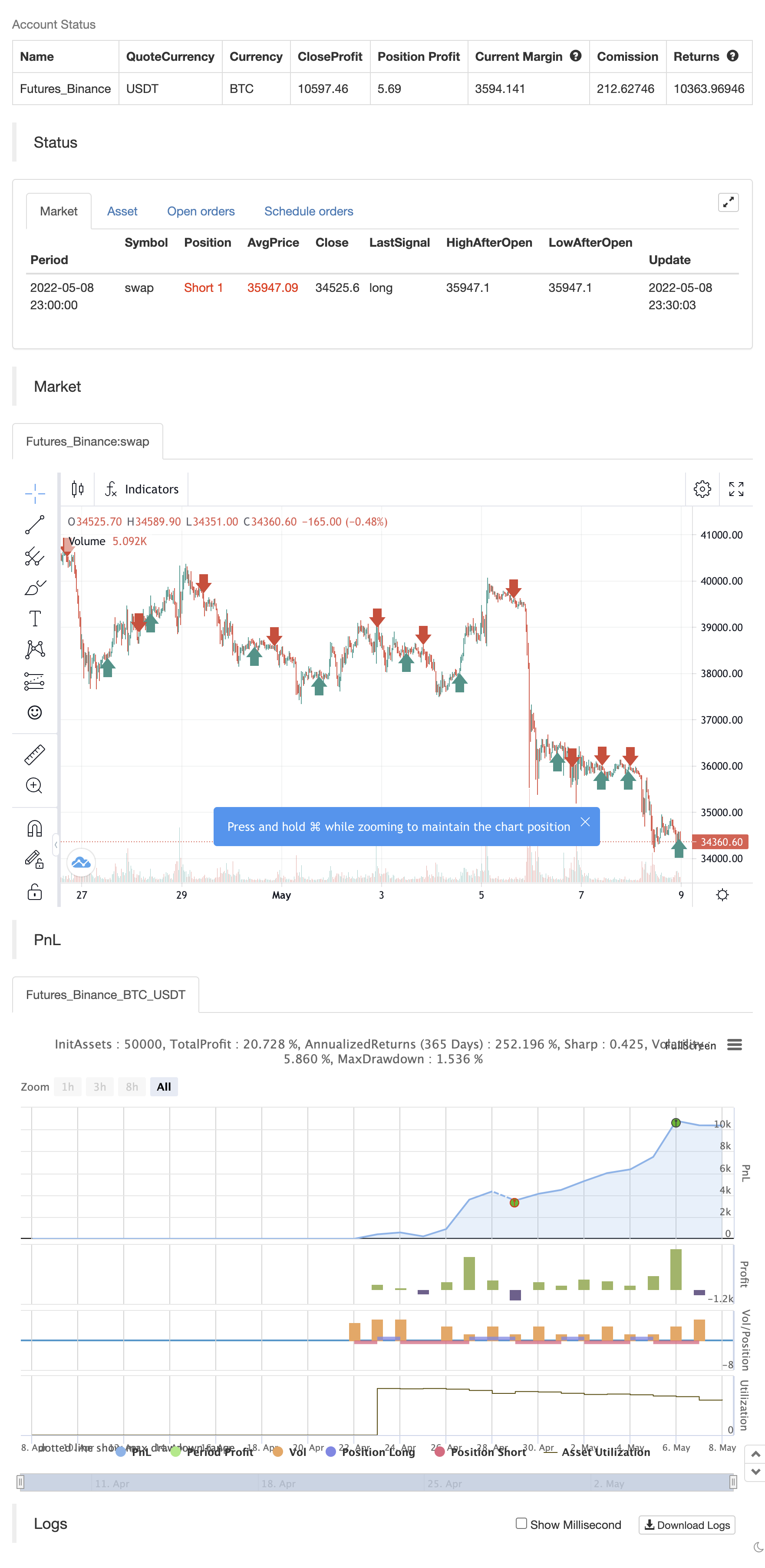# Momentum 2.0

Author: ChaoZhang, Date: 2022-05-10 10:24:44
Tags: 趋势

Momentum 2.0 is a normalized Momentum oscillator with a moving base-level. The oscillator value is normalized by its standard deviation, similar to the z-score technique. Instead of the zero level, the indicator uses the base-level calculated as the inverted long-term average value of the oscillator. Similar to the zero-level crossing signal used for the Momentum oscillator, our oscillator calculates the base level crossing signal. The moving base-level helps to reduce the number of false signals. In an uptrend the base-level is below zero, in a downtrend it is above it. This allows us to take into account the trend stability effect. In this case, to form a reversal signal, the oscillator must cross a lower value in an uptrend and a higher value in a downtrend.

HOW TO USE When the oscillator crosses above the base-level, it gives a bullish signal, when below it gives a bearish signal. The signals are displayed as green and red labels, respectively. The color of the histogram shows the current direction of the price momentum. Green indicates an upward move and red indicates a downward move. The blue line represents the base-level.

SETTINGS Oscillator Period - determines the period of the Momentum oscillator Base Level Period - determines the period used for long-term averaging when calculating the base-level and normalizing the oscillator

backtest```/*backtest
start: 2022-04-09 00:00:00
end: 2022-05-08 23:59:00
period: 30m
basePeriod: 15m
exchanges: [{"eid":"Futures_Binance","currency":"BTC_USDT"}]
*/

// This source code is subject to the terms of the Mozilla Public License 2.0 at https://mozilla.org/MPL/2.0/

//@version=5
indicator("Momentum 2.0", overlay = false)

source = close

// Script Inputs
window = input(defval=15, title="Oscillator Period")
base_level_window = input.int(defval=450, title="Base Level Period", minval=300)

// Calculate normalized and smoothed momentum oscillator
momentum = ta.mom(source, window)
momentum_normalized = ( momentum ) / ta.stdev(momentum, base_level_window)
momentum_smoothed = ta.linreg(momentum_normalized, 30,0)

// Calculated the base-level
momentum_base = -ta.ema(momentum_normalized,base_level_window)

// Calculate base-level cross signals
bullish = ta.crossover(momentum_smoothed, momentum_base)
bearish = ta.crossunder(momentum_smoothed, momentum_base)

if bullish
strategy.entry("Enter Long", strategy.long)
else if bearish
strategy.entry("Enter Short", strategy.short)
```

Related

More